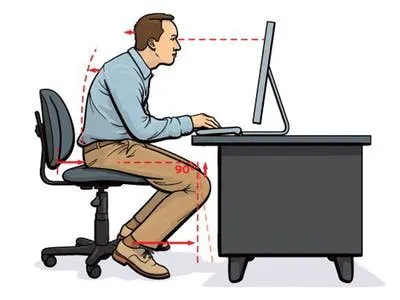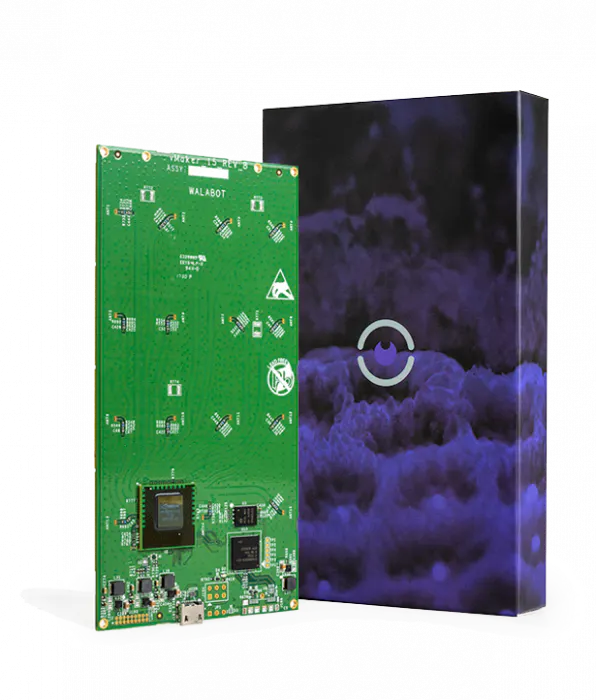# Posture Pal with Walabot

Prevent neck and back pain by monitoring your sitting posture with Walabot's distance sensor and an Android app.

IntermediateFull instructions provided8 hours1,993## Things used in this project

### Hardware componentsRaspberry Pi 3 Model B
×1Walabot Creator
×1

## Code

### server.py

Python
Flask server for HTTP long polling the Walabot distance on Raspberry Pi. Note: run first.
\$ python3 server.py
```#!/usr/local/env python
import json
import time

distance=0

@app.route('/set')
def set():
global distance
distance = request.args.get('distance')
return jsonify(distance)

@app.route('/status')
def status():
return Response(json.dumps({'status':distance}))

if __name__ == '__main__':
app.run(host='0.0.0.0', port=3000)
```

### distance.py

Python
Get distance to user from Walabot sensor on Raspberry Pi.
After running server.py:
\$ python3 distance.py
```from sys import platform
from os import system
import WalabotAPI
try:  # for Python 2
import Tkinter as tk
except ImportError:  # for Python 3
import tkinter as tk

MYPORT = 5002
import requests
import sys, time
from socket import *

class Walabot:

def __init__(self):
self.wlbt = WalabotAPI
self.wlbt.Init()
self.wlbt.SetSettingsFolder()
self.isConnected = False
self.isTargets = False

self.rMin = self.WalabotParameter(self, "R     Min", 1, 1000, 10.0)
self.rMax = self.WalabotParameter(self, "R     Max", 1, 1000, 100.0)
self.rRes = self.WalabotParameter(self, "R     Res", 0.1, 10, 2.0)
self.tMax = self.WalabotParameter(self, "Theta Max", 1, 90, 20.0)
self.tRes = self.WalabotParameter(self, "Theta Res", 1, 10, 10.0)
self.pMax = self.WalabotParameter(self, "Phi   Max", 1, 90, 45.0)
self.pRes = self.WalabotParameter(self, "Phi   Res", 1, 10, 2.0)
self.thld = self.WalabotParameter(self, "Threshold", 0.1, 100, 15.0)
self.mti = self.WalabotParameterMTI(self)
self.parameters = (
self.rMin, self.rMax, self.rRes, self.tMax, self.tRes,
self.pMax, self.pRes, self.thld, self.mti)

class WalabotParameter(tk.Frame):
""" The frame that sets each Walabot parameter line.
"""

def __init__(self, master, varVal, minVal, maxVal, defaultVal):
""" Init the Labels (parameter name, min/max value) and entry.
"""
self.minVal, self.maxVal = minVal, maxVal
self.var = tk.StringVar()
self.var.set(defaultVal)
self.var.trace("w", lambda a, b, c, var=self.var: self.validate())
txt = "[{}, {}]".format(minVal, maxVal)

def validate(self):
""" Checks that the entered value is a valid number and between
the min/max values. Change the font color of the value to red
if False, else to black (normal).
"""
num = self.var.get()
try:
num = float(num)
if num < self.minVal or num > self.maxVal:
self.entry.config(fg="red")
return
self.entry.config(fg="gray1")
except ValueError:
self.entry.config(fg="red")
return

def get(self):
""" Returns the entry value as a float.
"""
return float(self.var.get())

def set(self, value):
""" Sets the entry value according to a given one.
"""
self.var.set(value)

def changeState(self, state):
""" Change the entry state according to a given one.
"""
self.entry.configure(state=state)

class WalabotParameterMTI(tk.Frame):
""" The frame that control the Walabot MTI parameter line.
"""
def __init__(self, master):
self.mtiVar = tk.IntVar()
self.mtiVar.set(0)

def get(self):
""" Returns the value of the pressed radiobutton.
"""
return self.mtiVar.get()

def set(self, value):
""" Sets the pressed radiobutton according to a given value.
"""
self.mtiVar.set(value)

def changeState(self, state):
""" Change the state of the radiobuttons according to a given one.
"""
self.true.configure(state=state)
self.false.configure(state=state)

def getParameters(self):
""" Return the values of all the parameters entries/radiobuttons.
"""
rParams = (self.rMin.get(), self.rMax.get(), self.rRes.get())
tParams = (-self.tMax.get(), self.tMax.get(), self.tRes.get())
pParams = (-self.pMax.get(), self.pMax.get(), self.pRes.get())
thldParam, mtiParam = self.thld.get(), self.mti.get()
return rParams, tParams, pParams, thldParam, mtiParam

def setParameters(self):
""" Set the arena Parameters according given ones.
"""
rParams = (self.rMin.get(), self.rMax.get(), self.rRes.get())
tParams = (-self.tMax.get(), self.tMax.get(), self.tRes.get())
pParams = (-self.pMax.get(), self.pMax.get(), self.pRes.get())
thldParam, mtiParam = self.thld.get(), self.mti.get()

self.wlbt.SetProfile(self.wlbt.PROF_SENSOR)
self.wlbt.SetArenaR(self.rMin.get(), self.rMax.get(), self.rRes.get())
self.wlbt.SetArenaTheta(-self.tMax.get(), self.tMax.get(), self.tRes.get())
self.wlbt.SetArenaPhi(-self.pMax.get(), self.pMax.get(), self.pRes.get())
self.wlbt.SetThreshold(thldParam)
self.wlbt.SetDynamicImageFilter(mtiParam)
self.wlbt.Start()
def connect(self):
try:
self.wlbt.ConnectAny()
self.isConnected = True
self.setParameters()
except self.wlbt.WalabotError as err:
if err.code != 19:  # 'WALABOT_INSTRUMENT_NOT_FOUND'
raise err

def start(self):
self.wlbt.Start()

def get_targets(self):
self.wlbt.Trigger()
return self.wlbt.GetSensorTargets()

def stop(self):
self.wlbt.Stop()

def disconnect(self):
self.wlbt.Disconnect()

def calibrate(self):
""" Calibrate the Walabot.
"""
self.wlbt.StartCalibration()
while self.wlbt.GetStatus() == self.wlbt.STATUS_CALIBRATING:
self.wlbt.Trigger()

def print_targets(targets):
system("cls" if platform == "win32" else "clear")  # clear the screen
distance = str(targets.zPosCm)
r = requests.get("http://localhost:3000/set?distance=" + distance)

print(targets.zPosCm)

def main():
root = tk.Tk()

wlbt = Walabot()
wlbt.connect()

if not wlbt.isConnected:
print("Not Connected")
else:
print("Connected")

while True:
print_targets(wlbt.get_targets())

if __name__ == '__main__':
main()
```

### (Optional) Raspberry Pi gist for cloning (includes server.py and distance.py)

On Rasbperry Pi with TKinter and Flask installed: \$ git clone https://gist.github.com/justinshenk/aa1e1eb7ceb87fd82f0b655b5ad20c8a \$ python3 server.py \$ python3 distance.py

### Android app for Walabot

Build app (Optional) - git clone https://github.com/justinshenk/Walabot-PosturePal

## Credits

### Justin Shenk

3 projects • 26 followers
Machine learning and computer vision research engineer with background in neuroscience.